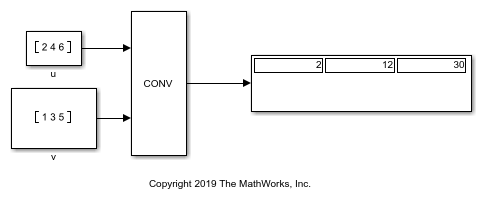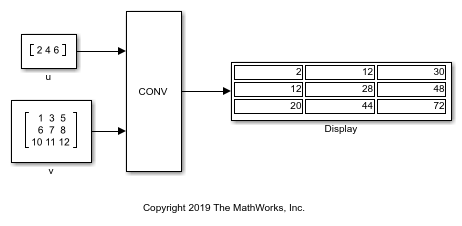# Convolution of Two Inputs

Using the Convolution block, convolve two input signals.

Open the `ex_convolution1.slx` model, which convolves two vectors.

For this model, the Convolution block returns a 1-by-3 vector. This is because both u and v are of the same shape and size.

```model1 = "ex_convolution1"; open_system(model1); sim(model1); ```Open the `ex_convolution2.slx` model, which convolves a vector with a matrix.

In this model, the Convolution block returns a 3-by-3 matrix. The two inputs can be convolved because they share the same last dimension, which becomes the size of the output's last dimension. The number of rows of the output is equal to the sum of the first dimension of the two inputs minus one. In this model that results in three rows, so the output is a 3-by-3 matrix.

```model2 = "ex_convolution2"; open_system(model2); sim(model2); ```When creating models that convolve N-D arrays, keep in mind that except for the first dimension, all other dimensions must be the same.